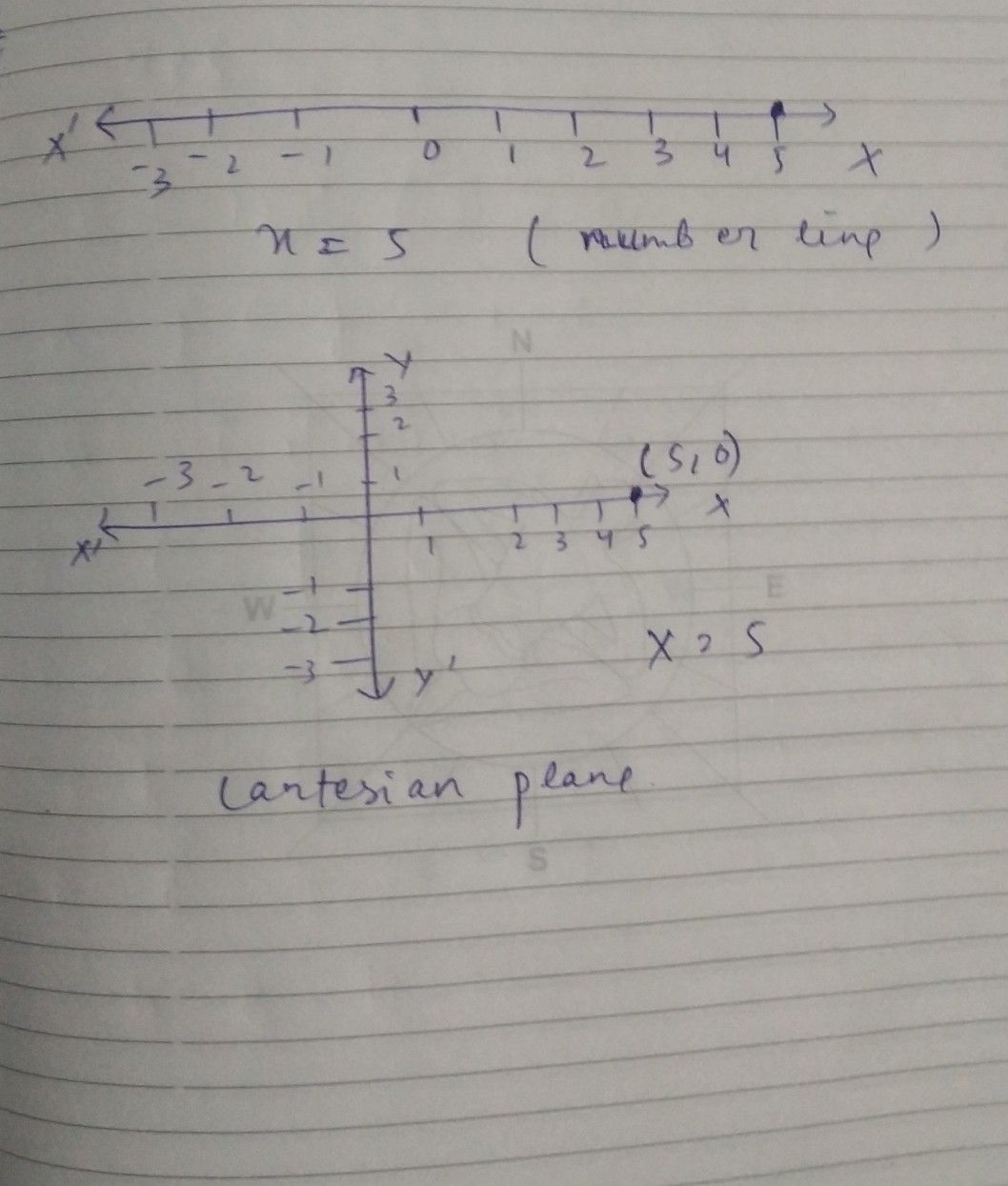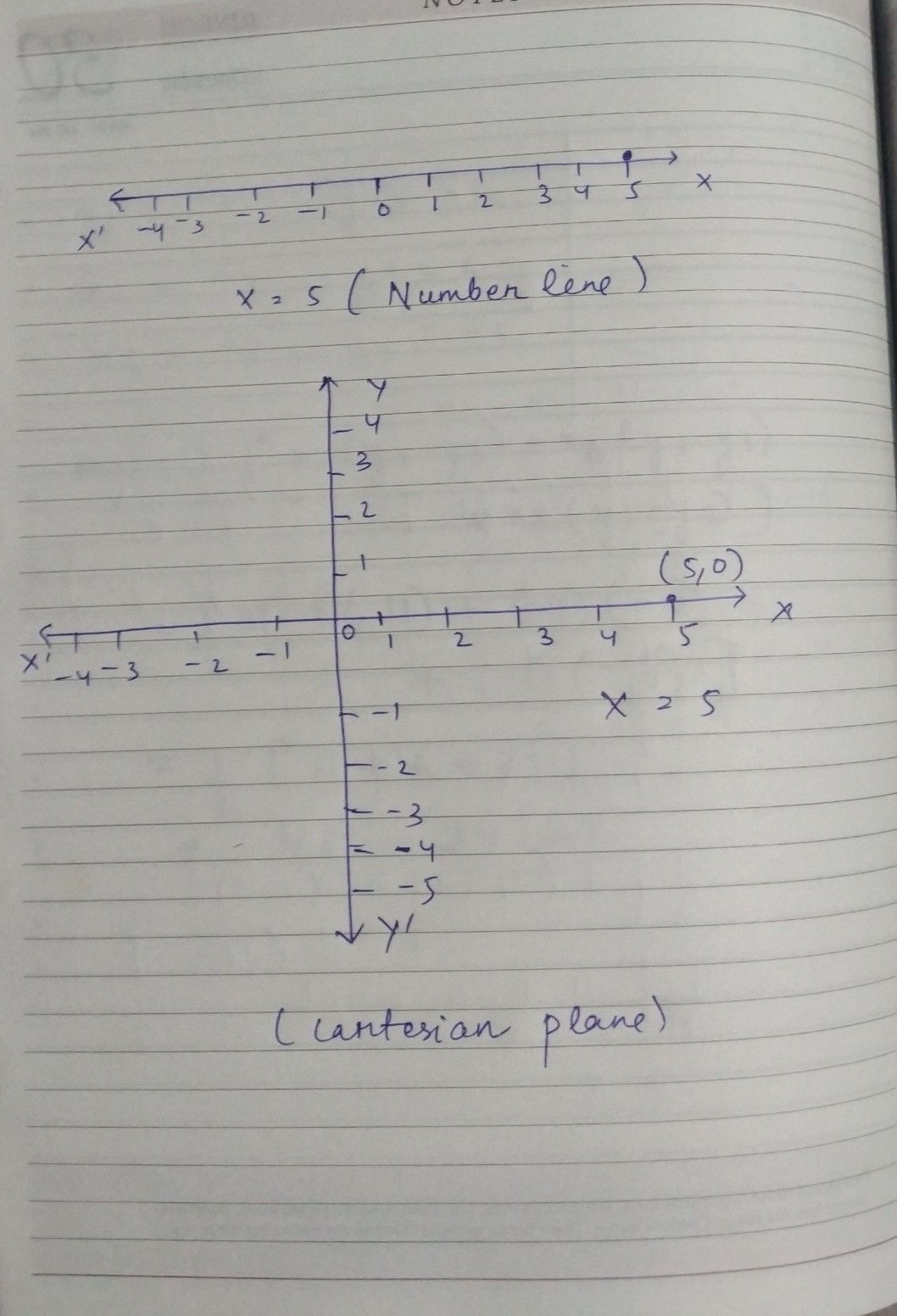Symbol
Problem$4$ Give the geometric representations of the following equations $\left(a\right)$ on the number line (b) $0n$ the Cartesian $0lane:$ $\times =5$ $\left(4\right)$
7th-9th grade
Calculus
Search count: 108
SolutionQanda teacher - ritsam18